# NCERT Solutions for Class 12 Physics chapter 6 Electromagnetic Induction

NCERT solutions for class 12 physics chapter 6 Electromagnetic Induction: In the chapter moving charges and magnetism you have studied that moving charges produces a magnetic field. The solutions of NCERT class 12 physics chapter 6 electromagnetic induction focus on the question based on the concept that the changing magnetic field can produce an emf across an electrical conductor. The equipment like an electric generator and the transformer work on this principle. In chapter 1 you have learnt about electric flux and in NCERT grade 12 chapter 6 electromagnetic induction you will learn about magnetic flux. The questions explained in the CBSE NCERT solutions for class 12 physics chapter 6 electromagnetic induction are based on the depth of understanding the concept. Many questions based on finding the directions of the magnetic field and current are explained in the NCERT solutions for class 12 physics chapter 6 electromagnetic induction which will be useful in exams. NCERT solutions are an important tool to perform better in the exams.

## NCERT solutions for class 12 physics chapter 6 electromagnetic induction exercise:To oppose the magnetic field current should flow in anti-clockwise, so the direction of the induced current is qrpq

## Q 6.1 (b)  Predict the direction of induced current in the situations described by the following Figs.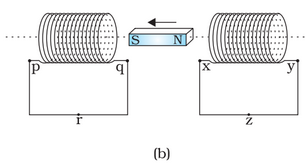Current in the wire in a way such that it opposes the change in flux through the loop. Here hence current will induce in the direction of p--->r--->q in the first coil and y--->z--->x in the second coil.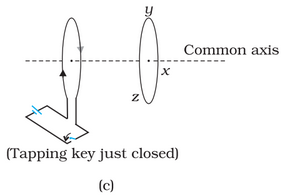When we close the key, the current will flow through the first loop and suddenly magnetic flux will flow through it such that magnetic rays will go from right to left of the first loop. Now, to oppose this change currently in the second loop will flow such that magnetic rays go from left to right which is the direction yzxy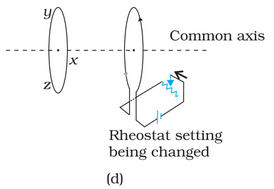hen we increase the resistance of the rheostat, the current will decrease which means flux will decrease so current will be induced to increase the flux through it. Flux will increase if current flows in xyzx.

On the other hand, if we decrease the resistance that will increase the current which means flux will be an increase, so current will induce to reduce the flux. Flux will be reduced if current goes in direction zyxz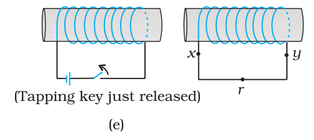As we release the tapping key current will induce to increase the flux. Flux will increase when current flows in direction xryx.

## Q 6.1 (f)  Predict the direction of induced current in the situations described by the following Fig (f)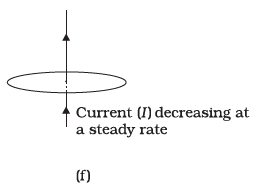The current will not induce as the magnetic field line are parallel to the plane. In other words, since flux through the loop is constant (zero in fact), there won't be any induction of the current.

A wire of irregular shape turning into a circular shape;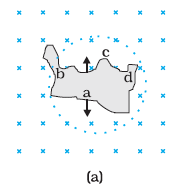By turning the wire from irregular shape to circle, we are increasing the area of the loop so flux will increase so current will induce in such a way that reduces the flux through it. By right-hand thumb rule direction of current is adcba.

A circular loop being deformed into a narrow straight wire.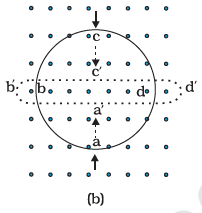Here, by changing shape, we are decreasing the area or decreasing the flux, so the current will induce in a manner such that it increases the flux. Since the magnetic field is coming out of the plane, the direction of the current will be adcba.

Given in a solenoid,

The number of turn per unit length :

loop area :

Current in the solenoid :

change in current :

change in time:

Now, the induced emf :

hence induced emf in the loop is .

Given:

Length of rectangular loop :

Width of the rectangular loop:

Area of the rectangular loop:

Strength of the magnetic field

The velocity of the loop :

Now,

a) Induced emf in long side wire of rectangle:

this emf will be induced till the loop gets out of the magnetic field, so

time for which emf will induce :

Hence a  emf will be induced for 2 seconds.

b) Induced emf when we move along the width of the rectangle:

time for which emf will induce :

Hence a  emf will induce for 8 seconds.

Given

length of metallic rod :

Angular frequency of rotation :

Magnetic field (which is uniform)

Velocity: here velocity at each point of the rod is different. one end of the rod is having zero velocity and another end is having velocity . and hence we take the average velocity of the rod so,

Now,

Induce emf

Hence emf developed is 100V.

Given

The radius of the circular loop

Number of turns

Flux through each turn

Flux through N turn

Induce emf:

Now,

maximum induced emf (when sin function will be maximum)

Average induced emf

as the average value of sin function is zero,

Maximum current when resistance  of the loop is .

Power loss :

Here, power is getting lost as emf is induced and emf is inducing because we are MOVING the conductor in the magnetic field. Hence external force through which we are rotating is the source of this power.

What is the instantaneous value of the emf induced in the wire?

Given

Length of the wire

Speed of the wire

The magnetic field of the earth

Now,

The instantaneous value of induced emf :

Hence instantaneous emf induce is .

What is the direction of the emf?

If we apply the Flemings right-hand rule, we see that the direction of induced emf is from west to east.

Which end of the wire is at the higher electrical potential?

The eastern wire will be at the higher potential end.

Given

Initial current

Final current

Change in time

Average emf

Now,

As we know, in an inductor

Hence self-inductance of the circuit is 4H.

## Q6.9  A pair of adjacent coils has a mutual inductance of . If the current in one coil changes from  to in , what is the change of flux linkage with the other coil?

Given

Mutual inductance between two coils:

Currents in a coil:

Change in current:

The time taken for the change

The relation between emf and mutual inductance:

Hence, the change in flux in the coil is .

Given

Speed of the plane:

Earth's magnetic field at that location:

The angle of dip that is angle made with horizontal by earth magnetic field:

Length of the wings

Now, Since the only the vertical component of the magnetic field will cut the wings of plane perpendicularly, only those will help in inducing emf.

The vertical component of the earth's magnetic field :

So now, Induce emf :

Hence voltage difference developed between the ends of the wing is  3.125V.

## NCERT solutions for class 12 physics chapter 6 electromagnetic induction additional exercise:

Given,

Area of the rectangular loop which is held still:

The resistance of the loop:

The initial value of the magnetic field :

Rate of decreasing of this magnetic field:

Induced emf in the loop :

Induced Current :

The power dissipated in the loop:

The external force which is responsible for changing the magnetic field is the actual source of this power.

Given,

Side of the square loop

Area of the loop:

The resistance of the loop:

The velocity of the loop in the positive x-direction

The gradient of the magnetic field in the negative x-direction

Rate of decrease of magnetic field intensity

Now, Here emf is being induced by means of both changing magnetic field with time and changing with space. So let us find out emf induced by both changing of space and time, individually.

Induced emf due to field changing with time:

Induced emf due to field changing with space:

Now, Total induced emf :

Total induced current :

Since the flux is decreasing, the induced current will try to increase the flux through the loop along the positive z-direction.

Given,

Area of search coil :

The resistance of coil and galvanometer

The number of turns in the coil:

Charge flowing in the coil

Now.

Induced emf in the search coil

Hence magnetic field strength for the magnet is 0.75T.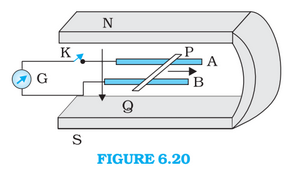Given

Length of the rod

Speed of the rod

Strength of the magnetic field

induced emf in the rod

Hence 9mV emf is induced and it is induced in a way such that P is positive and Q is negative.

## Q6.14 (b) Figure 6.20 shows a metal rod   resting on the smooth rails  and positioned between the poles of a permanent magnet. The rails, the rod, and the magnetic field are in three mutual perpendicular directions. A galvanometer  connects the rails through a switch . Length of the ,, resistance of the closed loop containing the . Assume the field to be uniform. Suppose K is open and the rod is moved with a speed of  in the direction shown. Give the polarity and magnitude of the induced emf.

(b)Is there an excess charge built up at the ends of the rods when  is open? What if  is closed?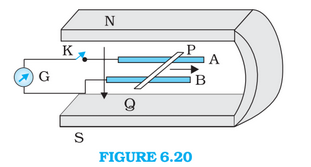Yes, there will be excess charge built up at the end of the rod when the key is open. This is because when we move the conductor in a magnetic field, the positive and negative charge particles will experience the force and move into the corners.

When we close the key these charged particles start moving in the closed loop and continuous current starts flowing.

(c) With K open and the rod moving uniformly, there is no net force on the electrons in the rod  even though they do experience magnetic force due to the motion of the rod. Explain.When the key K is open there is excess charge at both ends of the rod. this charged particle creates an electric field between both ends. This electric field exerts electrostatic force in the charged particles which cancel out the force due to magnetic force. That's why net force on a charged particle, in this case, is zero.

(d) What is the retarding force on the rod when K is closed?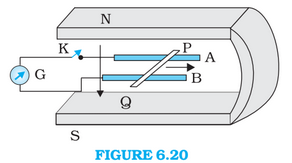Induced emf = 9mV (calculated in a part of this question)

The resistance of loop with rod = 9

Induced Current

Now,'

Force on the rod

Hence retarding force when k is closed is .

## Q6.14 (e) Figure 6.20 shows a metal rod   resting on the smooth rails  and positioned between the poles of a  permanent magnet. The rails, the rod, and the magnetic field are in three mutual perpendicular directions. A galvanometer  connects the rails through a switch . Length of the , , resistance of the closed loop containing the . Assume the field to be uniform. Suppose K is open and the rod is moved with a speed of  in the direction shown. Give the polarity and magnitude of the induced emf.

(e) How much power is required (by an external agent) to keep the rod moving at the same speed      when is closed? How much power is required when is open?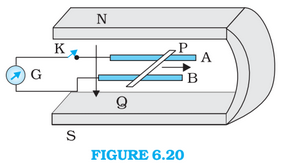Force on the rod

Speed of the rod

Power required to keep moving the rod at the same speed

Hence required power is 9mW.

When the key is open, no power is required to keep moving rod at the same speed.

## Q6.14 (f) Figure 6.20 shows a metal rod   resting on the smooth rails  and positioned between the poles of a permanent magnet. The rails, the rod, and the magnetic field are in three mutual perpendicular directions. A galvanometer  connects the rails through a switch . Length of the ,, resistance of the closed loop containing the . Assume the field to be uniform. Suppose K is open and the rod is moved with a speed of  in the direction shown. Give the polarity and magnitude of the induced emf

(f) How much power is dissipated as heat in the closed circuit? What is the source of this power?